# Biochemistry : Mixed Inhibition

## Example Questions

### Example Question #651 : Biochemistry

A mixed inhibitor was found to bind unequally to an enzyme and its enzyme-substrate complex. It has a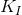of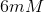for the enzyme, and aof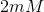for the enzyme-substrate complex.

If the inhibitor decreased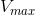by a factor of 3, what concentration of enzyme was used?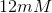The answer cannot be determined without knowing the change in the enzyme's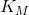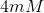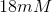Explanation:

Recall that the change inis only effected by the binding affinity to the enzyme substrate complex. We can represent the effect on theby this inhibitor by the following equation.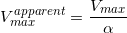Since the apparentdecreased by a factor of 3, we know that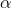is 3.

Solve for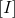in the following equation: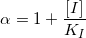Above,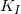is of the enzyme substrate complex, which is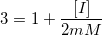Solve.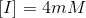### All Biochemistry Resources